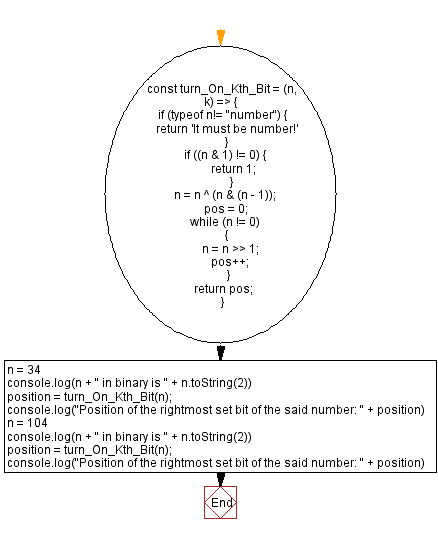# JavaScript - Position of the rightmost set bit of a number

## JavaScript Bit Manipulation: Exercise-12 with Solution

Write a JavaScript program to find the position of the rightmost set bit of a given number. The number 1 represents the set bits in a binary number.

Test Data:
(34) -> 2
Explanation:
34 in binary is 100010
Set bits in the 2nd position of the said binary format.
(104) -> 4
Explanation:
104 in binary is 1101000
Set bits in the 4th position of the said binary format.

Sample Solution:

JavaScript Code:

``````const turn_On_Kth_Bit = (n, k) => {
if (typeof n!= "number") {
return 'It must be number!'
}
if ((n & 1) != 0) {
return 1;
}
n = n ^ (n & (n - 1));
pos = 0;
while (n != 0)
{
n = n >> 1;
pos++;
}

return pos;
}

n = 34
console.log(n + " in binary is " + n.toString(2))
position = turn_On_Kth_Bit(n);
console.log("Position of the rightmost set bit of the said number: " + position)

n = 104
console.log(n + " in binary is " + n.toString(2))
position = turn_On_Kth_Bit(n);
console.log("Position of the rightmost set bit of the said number: " + position)
```
```

Sample Output:

```34 in binary is 100010
Position of the rightmost set bit of the said number: 2
104 in binary is 1101000
Position of the rightmost set bit of the said number: 4
```

Flowchart:Live Demo:

See the Pen javascript-bit-manipulation-exercise-12 by w3resource (@w3resource) on CodePen.

* To run the code mouse over on Result panel and click on 'RERUN' button.*

Improve this sample solution and post your code through Disqus

What is the difficulty level of this exercise?

Test your Programming skills with w3resource's quiz.

﻿